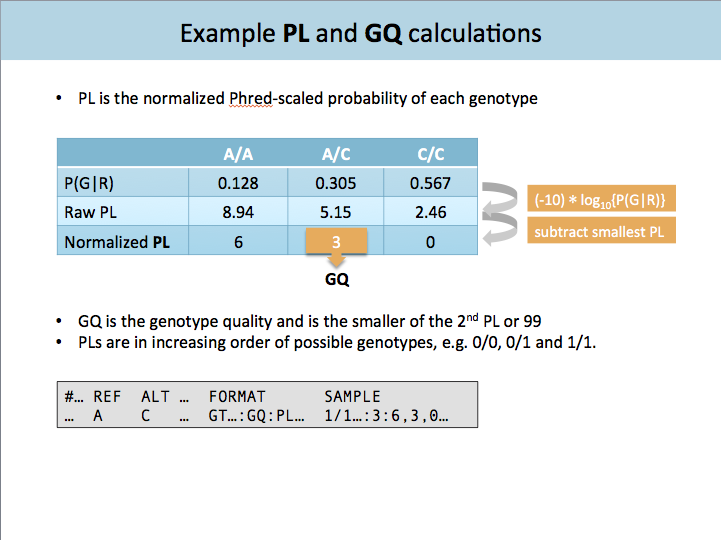#### Test-drive the GATK tools and Best Practices pipelines on Terra

Check out this blog post to learn how you can get started with GATK and try out the pipelines in preconfigured workspaces (with a user-friendly interface!) without having to install anything.

# Is PL actually a probability ratio not a likelihood? And consequences for GQ

I read the methods section Math notes: how PL is calculated in HaplotypeCaller which says that PL is based on the probability of the genotype given the data. Does this mean that it includes the product of the genotype likelihood and the prior probability of the genotype and therefore is it actually the (unnormalised) posterior probability not a likelihood? (I realise that the prior is by default flat but that it can be altered).

It then describes GQ as the ratio of the probability of the second-most probable genotype to the called genotype (if these are probabilities). Can you please explain how this equates to the probability that the genotype as been wrongly called given that the site is variant (from the VCF format specification). It doesn't take into account the probabilities of other possible genotypes and I don't understand how it is conditional on the site being variant. As an example, what about when the second-most probable genotype is homozygous reference.

Thanks for any help in understanding this - I am teaching it to students so what to check my understanding

## Answers

• Thank you for your answer shlee. You didn't answer the part about whether GQ is how it is defined in the VCF format. I don't see how it is conditioned on the site being variant. Could you please look at that part again?

• CambridgeMember, Broadie ✭✭✭✭✭

To clarify, here's a slide from a HaplotypeCaller presentation that uses toy values to simply illustrate the calculations:We log 10 transform the probability and multiply by -10 to obtain raw PLs (middle row). We then subtract the smallest PL from each raw PL so that the most likely genotype's PL is zero (last row). The distance to the next most likely genotype is the next most likely PL. The genotype quality (GQ) captures this distance. That is, the GQ is the PL of the next most likely, capped at 99.

Remember @Lucy_gen , low GQ does not necessarily mean a bad variant call. You can have a good variant call with a low GQ. That is, we can be sure a site is not hom-ref, but not be sure whether it is het or hom-var.

• Hi Shlee,

To be more specific, I expected that the GQ should be calculated as something like (1-posterior probabilty of called genotype)/(1-10^-QUAL/10) i.e. the probability that the genotype call is wrong divided by the probability that the site is variant. I think in the past GATK did have a calculation more like this, which seems to be closer to the definition in SAM format.

• Broad InstituteMember, Broadie, Moderator admin

@Lucy_gen
Hi,

I think this article and this article should help.

-Sheila

Sign In or Register to comment.